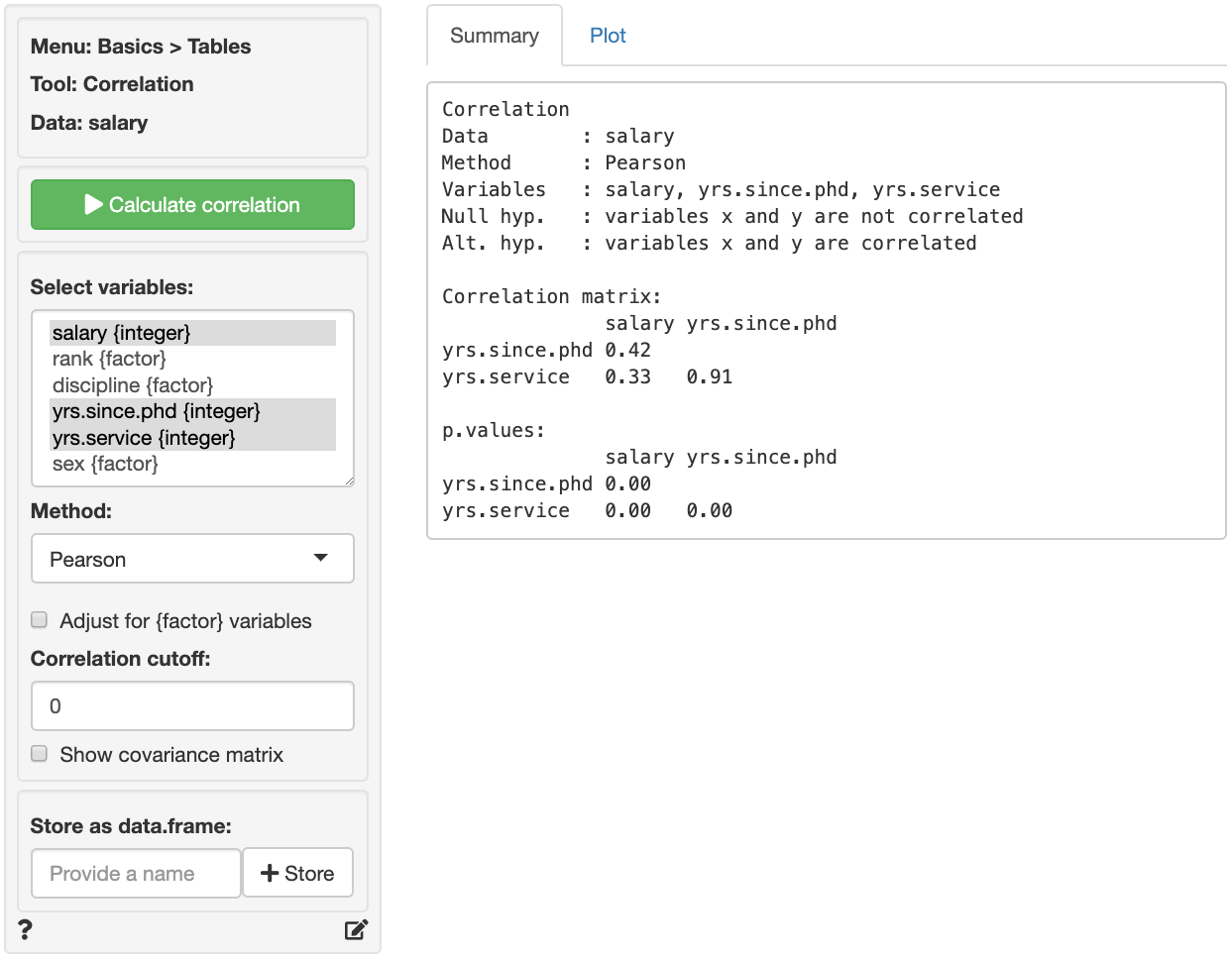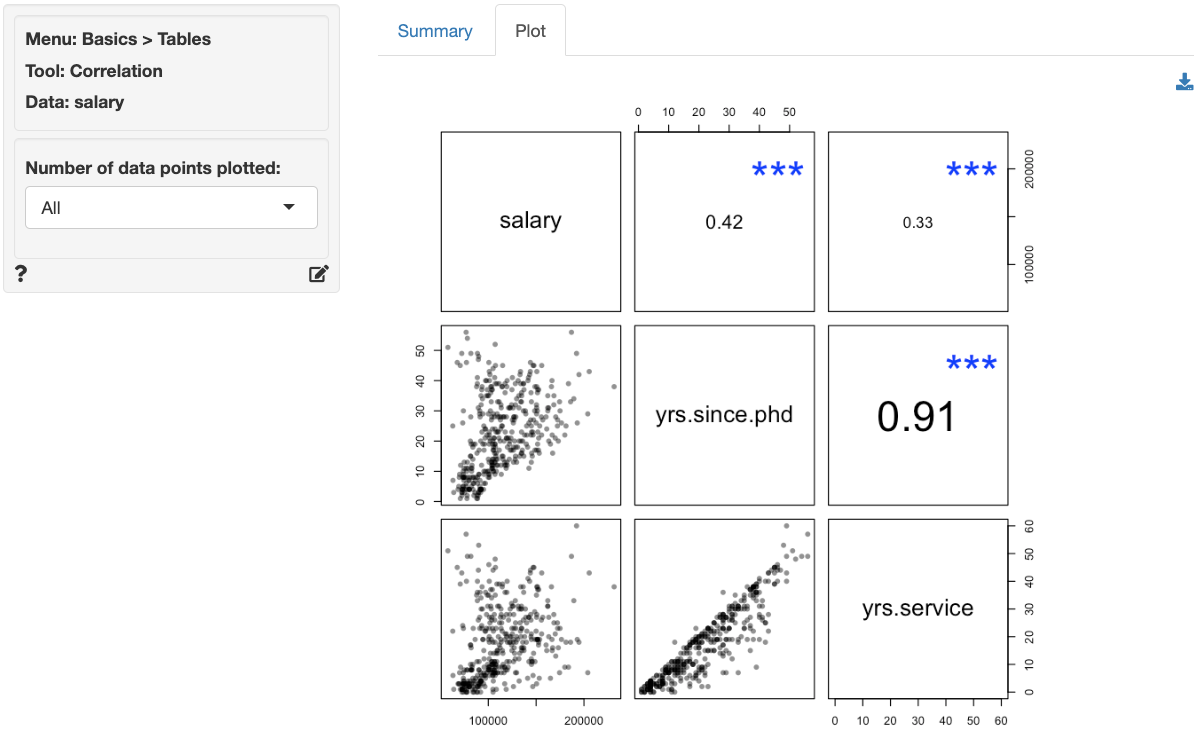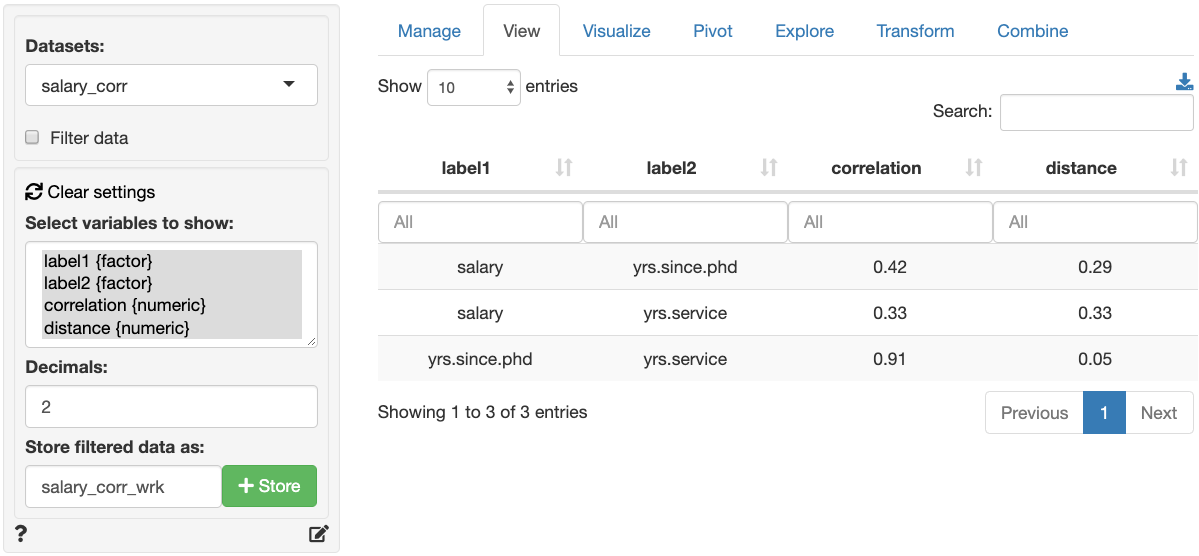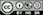How correlated are the variables in the data?

Create a correlation matrix of the selected variables. Correlations and p.values are provided for each variable pair. To show only those correlations above a certain (absolute) level, use the correlation cutoff box.

Note: Correlations can be calculated for variables of type numeric, integer, date, and factor. When variables of type factor are included the Adjust for {factor} variables box should be checked. When correlations are estimated with adjustment, variables that are of type factor will be treated as (ordinal) categorical variables and all other variables will be treated as continuous.A visual representation of the correlation matrix is provided in the Plot tab. Note that scatter plots in the graph at most 1,000 data points by default. To generate scatter plots that use all observations use plot(result, n = -1) in Report > Rmd.

Stars shown in the Plot tab are interpreted as:

• p.value between 0 and 0.001: ***
• p.value between 0.001 and 0.01: **
• p.value between 0.01 and 0.05: *
• p.value between 0.05 and 0.1: .The font-size used in the plot is proportional to the size and significance of the correlation between two variables.

### Method

Select the method to use to calculate correlations. The most common method is Pearson. See Wikipedia for details.

### Correlation cutoff

To show only correlations above a certain value choose a non-zero value in the numeric input between 0 and 1 (e.g., 0.15).

### Covariance matrix

Although we generally use the correlation matrix, you can also show the covariance matrix by checking the Show covariance matrix box.

## Store as data.frame

The correlation matrix can be stored as a data.frame by (1) providing a name for the new data set and (2) clicking on the Store button. The new data sets will the estimated correlation for each variable pair and a distance measure that is calculated as follows: distance = 0.5 * (1 - correlation). This measure will be equal to 1 when the correlation between two variable is equal to -1 and equal to 0 when the correlation between two variables is equal to 1. For an example of what such a dataset would look like, see the screenshot below of the Data > View tab. Data sets with this structure can be used as input to create a (dis)similarity based map by using Multivariate > (Dis)similarity.### Report > Rmd

Add code to Report > Rmd to (re)create the analysis by clicking the icon on the bottom left of your screen or by pressing ALT-enter on your keyboard.

By default the correlation plot samples 1,000 data points. To include all data points use plot(result, n = -1) To add, for example, a title to the plot use title(main = "Correlation plot\n\n"). See the R graphics documentation for additional information.

### R-functions

For an overview of related R-functions used by Radiant to evaluate correlations see Basics > Tables.

The key function from the psych package used in the correlation tool is corr.test.

© Vincent Nijs (2019)